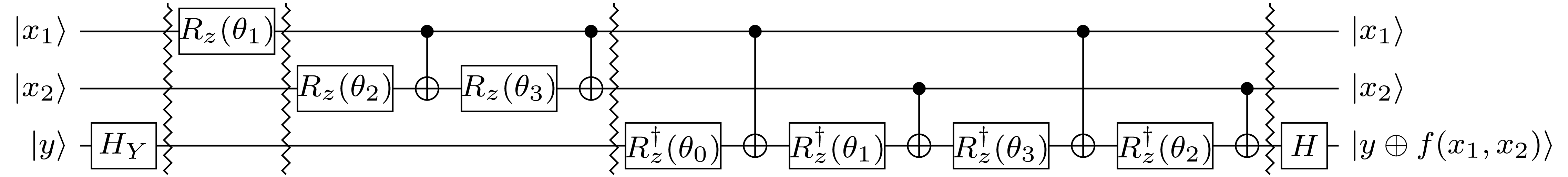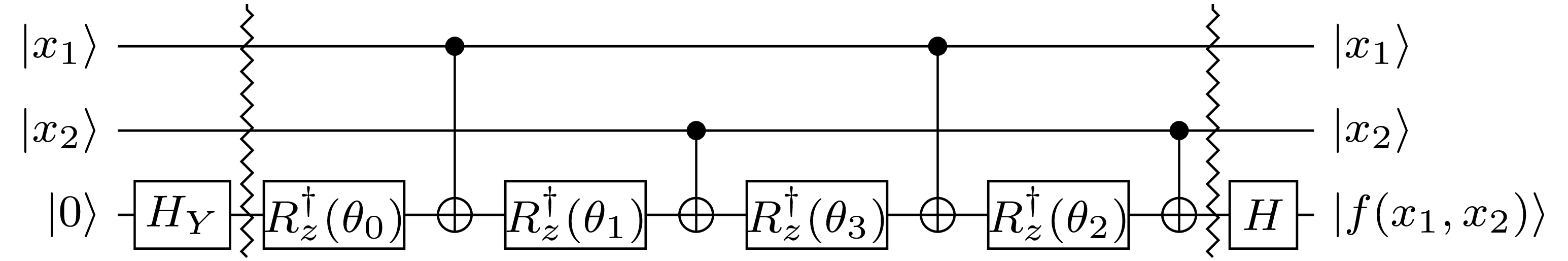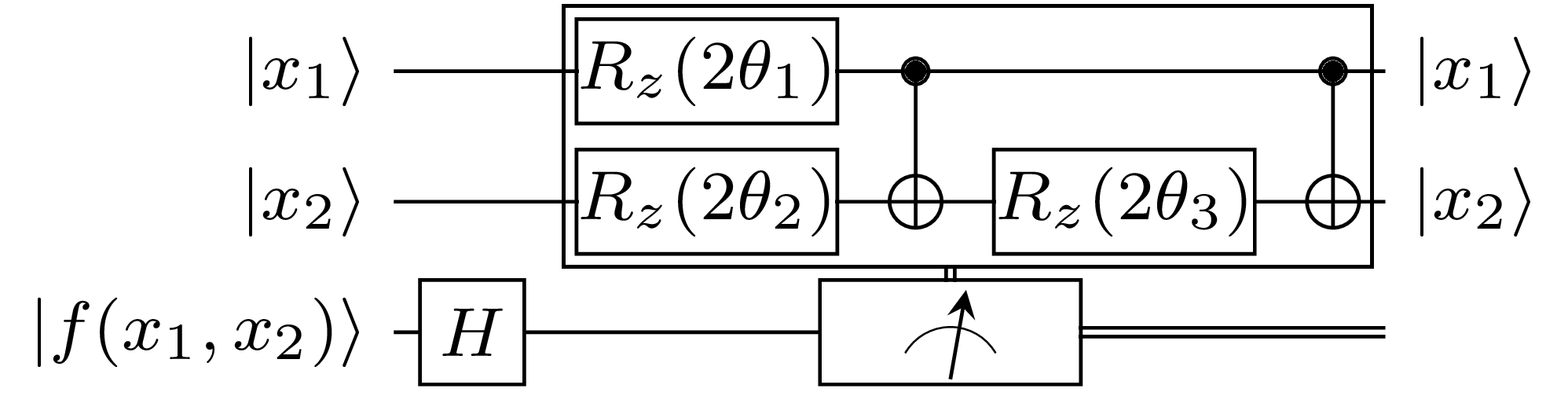# Quantum Oracle Circuits and the Christmas Tree Pattern

Blog article by Mathias Soeken from December 19, 2018, written for the Q# Advent Calendar

Donald E. Knuth introduced the Christmas tree pattern sixteen years ago in his ninth annual Christmas tree lecture at Stanford University, and he has made it part of Section 7.2.1.6 in his famous multi-volume work The Art of Computer Programming. The recursive definition of the Christmas tree pattern goes as follows. The Christmas tree pattern of order $$1$$ is a single row ‘$$0 \; 1$$’. One obtains the Christmas tree pattern of order $$n+1$$ by taking each row of elements ‘$$\sigma_1\; \sigma_2\; \ldots \sigma_s$$’ in the pattern of order $$n$$ and replace it by the following two rows: $$\begin{array}{ccccc} & \sigma_20 & \ldots & \sigma_s0 & \\ \sigma_10 & \sigma_11 & \ldots & \sigma_{s-1}1 & \sigma_s1\end{array}$$ The first of these two rows is omitted when $$s = 1$$. The Christmas tree pattern of orders $$2$$ to $$4$$ are:

 $$\begin{array}{ccc} & 10 & \\ 00 & 01 & 11 \end{array}$$ $$\begin{array}{ccc} & 100 & 101 & \\ & 010 & 110 & \\ 000 & 001 & 011 & 111 \end{array}$$ $$\begin{array}{ccc} & & 1010 & & \\ & 1000 & 1001 & 1011 & \\ & & 1100 & & \\ & 0100 & 0101 & 1101 & \\ & 0010 & 0110 & 1110 & \\ 0000 & 0001 & 0011 & 0111 & 1111 \end{array}$$

Knuth describes many interesting properties of this pattern in his book. But one of the most apparent ones is that the pattern of order $$n$$ contains all $$2^n$$ bitstrings of length $$n$$, grouped into $$n+1$$ columns according to the number of $$1$$’s in the bitstring. For now the Christmas tree pattern is visualized in green, but because it's Christmas, we should decorate the tree! In this blog article, I will use the implementation complexity of quantum oracles to decide which decoration to put.

The Christmas tree pattern of order $$n$$ can be used to visualize all the input combinations of an $$n$$-variable Boolean function. Moreover, the Christmas tree pattern of order $$2^n$$ can be used to either visualize all the input combinations of an $$2^n$$-variable Boolean function, but also to visualize all $$n$$-variable Boolean functions themselves. For example, the Christmas tree pattern of order $$4$$ contains all 16 2-variable Boolean functions.

#### Quantum oracles

The central quantum operations in this blog article are quantum oracle funtions $$U_f : |x_1x_2\ldots x_n\rangle|y\rangle \mapsto |x_1x_2\ldots x_n\rangle|y \oplus f(x_1, x_2, \dots, x_n)\rangle$$ implemented in terms of quantum circuits using the Hadamard gate, CNOT gates, and arbitrary Z-rotation gates. The following general circuit construction is based on the work by Schuch and Siewert and Welch et al, and here illustrated for a generic 2-variable Boolean oracle function $$f(x_1, x_2)$$.The nice property about this construction is that it can be used to find a circuit for each of the 16 possible functions $$f$$, by only changing the values for the rotation angles $$\theta_0, \dots, \theta_3$$. Before we discuss how to determine these values, I'd like to point out the recursive structure of the construction: If we leave out the surrounding Hadamard gate $$H$$ and $$Y$$-Hadamard gate $$H_Y = SH$$, there are three compartments $$1, 2, 3$$, separated by barrier lines, one compartment for each qubit. Let's for simplicity define $$x_3 = y$$. Then, the CNOT gates in compartment $$i$$ prepare all linear functions that include $$x_i$$ but do not include a variable with a larger index. All CNOT gates in compartment $$i$$ have the target on qubit $$i$$ and controls on qubits with a smaller index. The first compartment does not need a CNOT gate, since it has $$x_1$$ on the first qubit. The second compartment computes $$x_2 \oplus x_1$$, and then applies the CNOT gate again to revert the state of the second qubit back to $$x_2$$. Finally, CNOT gates in the third compartment are used to compute $$x_3 \oplus x_1$$, $$x_3 \oplus x_2 \oplus x_1$$, and $$x_3 \oplus x_2$$. The controls of the CNOT gates are chosen such that the control patterns are generated using a Gray code sequence—that minimizes the number of CNOT gates! Extending this circuit to a 4-qubit quantum circuit implementing oracles with 3 input variables is easy: one just needs to add a fourth compartment with 8 CNOT gates that add all linear functions that include $$x_4$$.

That explains the CNOT gates, but what about the angles $$\theta_j$$? These can be computed from the spectral coefficients $$s_j$$ of the oracle function $$f(x_1, x_2)$$. We obtain the spectral coefficients by applying the Hadamard transform $$H_2 = \left(\begin{smallmatrix} 1 & \hfil 1 \\ 1 & -1 \end{smallmatrix}\right) \otimes \left(\begin{smallmatrix} 1 & \hfil 1 \\ 1 & -1 \end{smallmatrix}\right)$$ to the truth table of $$f(x_1, x_2)$$ in $$\{-1,1\}$$-encoding. This encoding simply replaces every 0 with a 1, and every 1 with a -1. In other words, we have $$f_{x_2x_1} = (-1)^{f(x_1, x_2)}$$.

$$\left[ \begin{array}{rrrr} 1 & 1 & 1 & 1 \\ 1 & -1 & 1 & -1 \\ 1 & 1 & -1 & -1 \\ 1 & -1 & -1 & 1 \end{array} \right] \left[ \begin{array}{c} f_{00} \\ f_{01} \\ f_{10} \\ f_{11} \\ \end{array} \right] = \left[ \begin{array}{c} s_0 \\ s_1 \\ s_2 \\ s_3 \\ \end{array} \right]$$

By setting $$\theta_j = \frac{\pi s_j}{2^3}$$, the above circuit implements the oracle function. For example, if $$f(x_1, x_2) = x_1 \land x_2$$, then $$s_0 = s_1 = s_2 = 2$$, and $$s_3 = -2$$, and therefore $$\theta_0 = \theta_1 = \theta_2 = \frac{\pi}{4}$$ and $$\theta_3 = -\frac{\pi}{4}$$, hence, resulting in the well-known circuit construction with 7 $$T$$ and $$T^\dagger$$ gates. (Side remark: If $$|y\rangle = |0\rangle$$, then the circuit can be simplified by removing all but the last compartment. This generalizes Craig Gidney's construction for the Toffoli gate.)

#### Implementation in Q#

I have implemented the above algorithm for oracle synthesis in Q#. The complete source code is on Github, but the main operation is printed below. The first two lines in the operation Oracle compute the $$\{1,-1\}$$ value vector for the oracle function, as well as the spectral coefficients by applying the Hadamard transform in an efficient way using Yates's method. The Extend method extends the spectral coefficients by their inverses as described in the example above.

// First in tuple is value in GrayCode sequence
// Second in tuple is position to change in current value to get next one
//   Ex: GrayCode(2) = [(0, 0);(1, 1);(3, 0);(2, 1)]
function GrayCode(n : Int) : (Int, Int)[] {
// ...
}

operation Oracle(func : Int, controls : Qubit[], target : Qubit) : Unit {
let vars = Length(controls);
let table = Encode(TruthTable(func, vars));

let qubits = controls + [target];

HY(target);

for (i in 0..vars) {
let start = 1 <<< i;
let code = GrayCode(i);
for (j in 0..Length(code) - 1) {
let (offset, ctrl) = code[j];
RFrac(PauliZ, -spectrum[start + offset], vars + 2, qubits[i]);
if (i != 0) {
CNOT(qubits[ctrl], qubits[i]);
}
}
}

H(target);
}

The RFrac operation implements the rotation gates, and the CNOT operation implements the CNOT gate, which does not need to be applied in the first compartment.

#### Coloring the Christmas Tree

Let's apply the above oracle synthesis to all 3-input Boolean functions. Of course, each of the 256 functions has a different spectrum with 8 coefficients. But if we consider the absolute values of the coefficients and sort them, then all functions will fall into one of the following 3 classes:

$$0\, 0\, 0\, 0\, 0\, 0\, 0\, 8, \quad 0\, 0\, 0\, 0\, 4\, 4\, 4\, 4, \quad 2\, 2\, 2\, 2\, 2\, 2\, 2\, 6$$

The most narrow rotation angle in the first class is an $$S$$ gate, in the second class a $$T$$ gate, and in the third class a $$\sqrt{T}$$ gate. Let's decorate the Christmas tree of order 8, by coloring each function from the first class in green, from the second class in yellow, and from the third class in red. Merry Christmas!

 10101010 10101000 10101001 10101011 10101100 10100100 10100101 10101101 10100010 10100110 10101110 10100000 10100001 10100011 10100111 10101111 10110010 10110000 10110001 10110011 10110100 10010100 10010101 10110101 10010010 10010110 10110110 10010000 10010001 10010011 10010111 10110111 10111000 10011000 10011001 10111001 10001010 10011010 10111010 10001000 10001001 10001011 10011011 10111011 10001100 10011100 10111100 10000100 10000101 10001101 10011101 10111101 10000010 10000110 10001110 10011110 10111110 10000000 10000001 10000011 10000111 10001111 10011111 10111111 11001010 11001000 11001001 11001011 11001100 11000100 11000101 11001101 11000010 11000110 11001110 11000000 11000001 11000011 11000111 11001111 11010010 11010000 11010001 11010011 11010100 01010100 01010101 11010101 01010010 01010110 11010110 01010000 01010001 01010011 01010111 11010111 11011000 01011000 01011001 11011001 01001010 01011010 11011010 01001000 01001001 01001011 01011011 11011011 01001100 01011100 11011100 01000100 01000101 01001101 01011101 11011101 01000010 01000110 01001110 01011110 11011110 01000000 01000001 01000011 01000111 01001111 01011111 11011111 11100010 11100000 11100001 11100011 11100100 01100100 01100101 11100101 01100010 01100110 11100110 01100000 01100001 01100011 01100111 11100111 11101000 01101000 01101001 11101001 00101010 01101010 11101010 00101000 00101001 00101011 01101011 11101011 00101100 01101100 11101100 00100100 00100101 00101101 01101101 11101101 00100010 00100110 00101110 01101110 11101110 00100000 00100001 00100011 00100111 00101111 01101111 11101111 11110000 01110000 01110001 11110001 00110010 01110010 11110010 00110000 00110001 00110011 01110011 11110011 00110100 01110100 11110100 00010100 00010101 00110101 01110101 11110101 00010010 00010110 00110110 01110110 11110110 00010000 00010001 00010011 00010111 00110111 01110111 11110111 00111000 01111000 11111000 00011000 00011001 00111001 01111001 11111001 00001010 00011010 00111010 01111010 11111010 00001000 00001001 00001011 00011011 00111011 01111011 11111011 00001100 00011100 00111100 01111100 11111100 00000100 00000101 00001101 00011101 00111101 01111101 11111101 00000010 00000110 00001110 00011110 00111110 01111110 11111110 00000000 00000001 00000011 00000111 00001111 00011111 00111111 01111111 11111111

#### Appendix

Above, it is mentioned that the technique generalizes to Craig Gidney's construction for the Toffoli gate. The technique applies to all Boolean functions with $$n$$ variables, but is illustrated for simplicity for $$n = 2$$. If the target qubit is initialized to $$|0\rangle$$, one only needs the last compartment, and can omit all other ones:When uncomputing the oracle function, i.e., we expect that the ancilla qubit is restored to 0, one can use the following circuit which uses all but the last compartments from the circuit. Also, all angles are multiplied by two:Both circuits can be implemented inside a single Q# operation using the adjoint keyword:

operation OracleAncilla(func : Int, controls : Qubit[], target : Qubit) : Unit {
body (...) {
let vars = Length(controls);
let table = Encode(TruthTable(func, vars));

HY(target);

let code = GrayCode(vars);
for (j in 0..Length(code) - 1) {
let (offset, ctrl) = code[j];
RFrac(PauliZ, spectrum[offset], vars + 2, target);
CNOT(controls[ctrl], target);
}

H(target);
}
let vars = Length(controls);
let table = Encode(TruthTable(func, vars));

H(target);
if (IsResultOne(M(target))) {
for (i in 0..vars - 1) {
let start = 1 <<< i;
let code = GrayCode(i);
for (j in 0..Length(code) - 1) {
let (offset, ctrl) = code[j];
RFrac(PauliZ, -spectrum[start + offset], vars + 1, controls[i]);
if (i != 0) {
CNOT(controls[ctrl], controls[i]);
}
}
}
Reset(target);
}
}
}